# More How to Win at Aptitude Tests# More How to Win at Aptitude Tests Liam Healy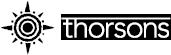# Contents

Welcome and well done. You have probably picked up this book for one of three reasons:

1 You, or someone you know, are about to take some aptitude tests and you think you may need a hand;

2 You are facing some choices regarding your own career and you want to find out what you are good at and what you are not so good at;

3 You have heard about aptitude tests and know that a lot of companies use them for recruitment or development purposes, so you want to brush up your skills – just in case.

The numerous test examples and detailed information in this book will help you prepare for test sessions, and give you an idea of what to expect during an actual test session, so reducing your anxiety about having to face what can be the most daunting part of a recruitment process. The reasons why companies use tests to make decisions and how they use them, are explained. Tests are very often followed by post-test feedback and interviews, and the best approaches to these are described.

## Practice Tests

In this book you will find examples of the most common types of test in use today, as well as examples of some of the less common. For every type of test, preparation and practice strategies are included, as well as detailed answers to the questions. For the most basic ability tests, through to graduate and managerial level reasoning and problem-solving tests, this book is likely to be your best single source for preparing for that all-important test.

With the increasing emphasis companies place on the ‘softer’ behavioural skills, a specially written personality test has also been included – you are more likely to face this type of test than any other. This will help give you a complete picture of what you are like as a person. Whatever type of test you are going to face, this book will show you what to expect.

It is only natural that when faced with an unknown task you will be nervous. This is true whether you are a school leaver applying for your first job, or a senior executive applying for your final post before retirement. Aptitude testing is used for more than just recruitment; for many companies it plays a pivotal role in development as well – in either case, knowing what to expect will enable you to produce a performance which is less hindered by nerves and which reflects more closely your actual skills and abilities.

## Handling the Selection Process

Being invited to sit a test (or tests) is only one part of the selection process. This book explains what tools an employer may use to assess the skills and abilities that they are interested in. The other types of selection tool you may also come across, such as assessment centres, interviews, work samples and job simulations, are also described.

Because most employers use structured interviews to validate the results of tests, the post-test interview is covered in detail. This includes advice on how to recover if you think your performance has been less than perfect!

Whatever you choose to do in your life – and it is your life and happiness which are important, not simply your career – this book gives you the chance to prepare yourself for some of the challenges you may face.

Good luck!

An aptitude test is one of a larger group of measures of psychological characteristics collectively known as ‘psychometric tests’. Overall, psychometric tests fall into one of two categories:

1 Tests of Typical Performance – which include things such as personality tests and interest questionnaires.

2 Tests of Maximum Performance – which include things such as aptitude and ability tests.

## TYPICAL AND MAXIMUM PERFORMANCE Typical Performance Test

A typical performance test looks at what you can typically do. This means measuring what you can do without any real effort or preparation.

## Personality questionnaires

The most common type of typical performance test is the personality questionnaire, but they can also be used to measure things such as work preferences and interests. Personality questionnaires usually contain items (or questions) in the form of:

Q I would rather go out and socialize with my friends than stay in and read a book.

A (choose one)

Agree □

Disagree □

Clearly, there is no ‘correct’ answer to this question. Assuming that a question measures a personal trait such as ‘enjoyment of social contact’, there is no particular meaning or value attached to either answer. In other words, neither answer is intrinsically good or bad. So, for instance, saying that a person scored 23 out of 56 on an assertiveness scale is probably meaningless, while saying that they were more assertive than average might have some relevance to their suitability for employment. Most typical performance tests, such as personality questionnaires, have no time limit, although, for organizational efficiency, some test supervisors may encourage you to complete them within a set time.

## Tests of interest and tests of motivation

There are two other types of typical performance test you may come across – tests of interest and tests of motivation. Tests of interest are rarely used in selection processes, being more commonly used in career development. Tests of motivation are just that – measures of what motivates a person. However, they are quite rare and very seldom used in selection, not least because no one can agree what exactly tests of motivation should be looking for.

## Maximum Performance Tests

A maximum performance test looks at what you can actually do when you are trying your best and this is exactly what an aptitude or ability test is. Look at the following from a numerical aptitude test:

123 × 456 = ?

Clearly, if you do not put effort into answering this question, you will not get the answer right. Your gut feeling might produce an answer of about 500,000. This is not the correct answer, and if you put this down as the answer you would be wrong. If you tried harder you might eventually come up with the correct answer (56,088).

So, with tests of maximum performance, the questions do have right and wrong answers and so the results can be interpreted numerically with greater ease than typical performance tests.

One of the things we know about intellectual ability is that people who are rated as being more intelligent than others may be so because they can solve problems more quickly. It follows that tests of maximum performance, such as aptitude tests, usually have strict time limits.

## ABILITY AND APTITUDE

If you sat a test that assessed your numerical skills, you may find that it would be called something like a ‘Numerical Aptitude’ test. Subsequently, you may sit a test that looks exactly the same but instead is called a ‘Numerical Ability’ test. They certainly look the same – but there is a difference.

In reality, they both measure numerical skills. Psychologists have known for a long time that intelligence, or intellectual ability, is composed of a number of separate facets. Generally, these areas are accepted as being linked to verbal, numerical, abstract, spatial and mechanical reasoning.

If we were only interested in, say, mechanical reasoning, we would refer to this very specific area as ‘aptitude’ or ‘specific ability’. Very often, a person’s scores on three tests – for example, verbal, numerical and abstract ability – are combined to produce an overall score. More rarely, because of the time involved, a test may have questions from all three.

When used in this combined way, a measure of a person’s overall general ability or general aptitude is obtained. Remember:

Ability = general aptitude or overall ability at verbal, numerical and abstract thinking.

Aptitude = specific ability, such as in verbal or numerical or abstract thinking.

This distinction is not so important for the average test taker, since you may simply be told to expect a test of numerical reasoning skill, so don’t worry too much about it.

It is important, though, not to confuse tests of ability and aptitude with tests of attainment. These assess what you have learnt, and are tests of knowledge not ability. Things like school or driving exams are tests of attainment rather than direct measures of ability.

## SPEED AND POWER TESTS

Speed and Power tests represent different styles of test, rather than measuring different aptitudes or abilities; specifically, they describe the nature of the test questions themselves.

In a speed test, the items tend to be quite small in scope, very specific and often not too difficult. With speed tests it is how many questions the test taker can answer correctly that is important. A numerical aptitude test with speed items could look something like this; you can see how each question is very narrowly confined in terms of content:

123 + 456 = ?

987 – 654 = ?

567 × 123 = ?

In the case of a power test, the approach is quite different and much more emphasis is placed on presenting the test taker with a smaller number of more difficult or complex questions.

A numerical aptitude test with power items may look something like:

Brian is 6 cm taller than Kate and Kate is 2 cm taller than William. William is 1,680 cm tall. Andrew is taller than all three but not as tall as Marjorie.

Brian is taller than Andrew

a) true

b) false

c) can’t tell

Brian is 1,692 cm tall

a) true

b) false

c) can’t tell

Typically, speed tests contain a lot more items than power tests although they often have the same approximate time limit. Power tests tend to be used more at the graduate, professional or managerial level since their expanded format allows for more work-related content to be addressed.

## APTITUDE AND REASONING ABILITY

Although reasoning ability does depend on some level of ‘raw’ or basic ability, aptitude and reasoning ability are two different things. Straightforward ability or aptitude is to do with the computational acts involved in solving a very specific test question – what you have to do is often clear, whether you can do it or not is quite a different matter. Reasoning ability is to do with knowing when to apply a certain computational act.

In the power and speed test examples above, the speed questions were to do with basic, raw numerical or mathematical ability. A very precise act of computation (for instance subtraction or multiplication) was clearly indicated by the format of the question. All you had to do was carry out the calculation.

With the power questions, it was not indicated which specific mathematical computation (for instance, addition or division) had to be carried out in order to reach a solution. The task you faced was to apply a more general problem solving approach – the problem being not ‘what is the answer?’ but ‘what do I have to do to work out how to reach the answer?’

Once you have established how to reach the answer, the final task itself is often a very simple process of mathematical calculation.

Brian is 1,692 cm tall

a) true

b) false

c) can’t tell

If William is 1,680 cm tall, Kate is 2 cm taller than William, and Brian is 6 cm taller than Kate then the answer must be:

1,680 cm + 2 cm + 6 cm = 1,688 cm.

So the statement that Brian is 1692 cm tall is false.

Notice how simple the mathematics was once we had worked out what to do. This is a good example of numerical reasoning ability or aptitude, rather than just straightforward numerical ability or aptitude. Furthermore, because the information is presented verbally, this is also a reasonable example of what verbal reasoning test items may look like. Power tests are more commonly found in the numerical and verbal domains but can also crop up in the mechanical, abstract and perceptual ability areas.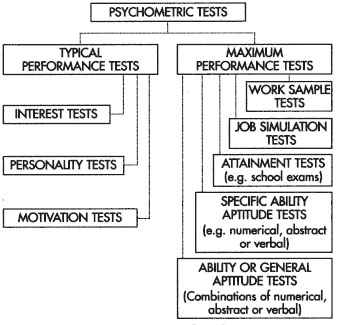## OTHER TYPES OF TEST

The other two commonest types of test are Work Samples and Job Simulations, although at first glance they do not look as we imagine tests to be.

A Work Sample test is exactly what its name implies – a sample of the work the candidate is required to do. These tend to be used widely in mechanical or practical areas of work. A common example is a typing test where the candidate has to type out some hand-written notes without making any errors within a given period of time. Another example is a fork-lift truck driver moving a set number of pallets from one place to another to within a certain degree of accuracy. If you are invited for a Work Sample test, the chances are you already have some skill in the area to begin with.

Job Simulations look similar to Work Samples and the two are often confused. However, whereas a Work Sample actually involves doing a sample of the activities carried out in the target job, a Job Simulation only simulates those activities involved in the target job.

If our fork-lift truck driver was inexperienced, it would clearly not be wise to put him or her into a fork-lift truck and see how little damage is done! It would be better to measure those abilities we know are involved in driving a fork-lift truck, such as hand to eye co-ordination, spatial awareness and so on, and then use the information obtained to infer how the person might perform in the job once they had been trained. Because of this, Job Simulations are often used when selecting people for training or when they have no direct experience in or specific knowledge of the job in question.

Another common type of Job Simulation is the so-called ‘In-basket’, or ‘In-tray’, which is used for many management-based selection activities. The In-tray simply involves taking the contents of a manager’s typical in-tray and working through them, making decisions as to what to do with each item as you go. These simulations are often used to assess organizational and decision-making ability, and are quite commonly used in management selection. However, you do need the contents of a real in-tray to base the exercise on and these contents can be both numerous and diverse – an entire in-basket could easily fill this book!

In addition, tests like In-trays are very difficult to score correctly because there is often no single correct way to deal with the items. Consequently, they tend to be followed up by a presentation from, or interview with, the candidate during which they explain how they worked their way through the in-tray, what criteria they used for decision making and how they justified their actions.

## HOW AND WHY COMPANIES USE APTITUDE TESTS Where Do Tests Fit into the Wider Selection Process?

The purpose of a selection system is to make a prediction about who will be successful at the job. It does this by gathering evidence about a candidate, weighing that evidence and then making a decision as to whether or not to offer them a position.

One thing that many candidates mistakenly believe is that the test constitutes the complete selection process. In fact, tests are very rarely used as a first stage in selection. Usually, the process begins long before a job is advertised and typically it involves the following steps.

## Job Analysis

This is carried out to determine the key tasks of the job. It can involve sophisticated analytical methods using data about several hundred job holders, or it can be done using ‘expert analysis’ which involves a group of individuals, who are very familiar with the job, sitting down and agreeing what the job involves. In cases where there are relatively few employees (which rules out large-scale statistical analysis) the latter method tends to be used.

## Producing the Job Description

This is a formal description of the tasks identified as a result of the job analysis.

## Producing the Person Specification

This often takes quite a lot of work. The key challenge for the organization here is to identify the knowledge, skills, attributes and abilities a person must possess if they are to be able to carry out the tasks described in the Job Description. Very often these characteristics, contained in the Person Specification, are classified as being either ‘essential’ or ‘desirable’.

## Identifying What the Success Criteria Will Be

This involves setting success criteria for future work performance for use in work appraisals. This stage is often overlooked, but it remains an important part of the process since it allows the organization to analyze how well their selection system and selection tools actually predicted work success.

## Choosing the Selection Tools

The selection tools are then chosen based on their effectiveness at measuring those characteristics described in the Person Specification. They may include interviews, tests, CVs, application forms, and individual or group exercises.

## Applying the Chosen Selection Tools

This is often done in several stages, with successful candidates at one stage being invited to participate in the next. Sometimes, candidates may be pre-selected based on qualifications or experience, and then invited to come along to an assessment day (sometimes known as an assessment centre), during which all of the tools are used.

## Weighing Evidence Gathered From the Selection Tools

Usually, no single assessor has the final say over any one candidate. In the case of assessment centres in particular, an observation and scoring system is devised which means that different assessors observe different candidates over the course of the day, and the ratings allocated to each candidate by each assessor are scored using a standardized scoring system. Conflicting evidence is discussed and an overall rating is agreed upon by the whole assessment team. This occurs after the assessments have been carried out and the candidates have gone home.

## Making a Selection Decision

This is based on the evidence that has emerged from the assessment process. The decision as to whether or not to offer someone a job is seldom solely based upon the need to hire someone, rather it is based upon the evidence. It is not uncommon for an organization not to hire any of the candidates they have seen, especially if all of the candidates have weaknesses in one or more ‘essential’ areas of the person specification.

The final decision can be based on a simple test score ‘cut-off’ as a first stage (where test scores are clearly related to job performance). A more sophisticated process might involve ‘regression’ or ‘factor analysis’, and a ‘multi-stage actuarial decision process’. But these are in the realms of advanced selection theory and beyond the scope of this book.

There is no use in worrying about it – you can’t influence the decision making process, mainly because you will not be there when it goes on! Do bear in mind that if this process has been gone through thoroughly by the organization, you can take some comfort from the fact that the selection or recruitment process and decision-making mechanism are based on evidence and have been designed to be as objective and error free as possible. The fact that an organization uses tests should be viewed as a source of comfort rather than dread.

## WILL I ONLY COME ACROSS TESTS WHEN I APPLY FOR A JOB?

The answer to this question is probably ‘No’. When one considers the type of information a test produces, it is clear that the information can be broadly classified into two areas:

1 This person has the ability that is required.

2 This person does not have the ability that is required.

When looked at like this, the outcome from a test can also be used very effectively in development. This is particularly the case with the ‘softer’ behavioural skills which personality tests assess.

Tests are also used extensively in Career Development work, often in conjunction with a Personal or Career Development Plan.

## FINALLY, THE TESTS!

In the following chapters we will go through example tests. For some types of test there is quite a bit of background involved. This is because areas such as numerical ability can cover a wide range of information and are applicable to many different types of job. Numerical ability testing is one area people worry about more than any other, so there is more space given to this. Other areas, such as mechanical reasoning, are more straightforward, more self-explanatory. The main categories of aptitude tests covered are:

Basic Numerical Ability – These tests measure basic mathematical ability.

Numerical Reasoning – These tests measure higher-level or more complex problem solving where the problems are numerical in nature.

Basic Verbal Ability – These tests measure basic verbal abilities such as spelling and comprehension.

Verbal Reasoning – These tests measure higher-level or more complex problem solving where the problems are verbal in nature. They are sometimes known as ‘critical thinking’ tests.

Abstract Reasoning – These tests measure problem solving ability in a context-free environment and are largely based on non-verbal and non-numerical questions.

Spatial Reasoning – These tests measure the ability to perform operations such as rotation or combining different features of two- and three-dimensional objects in one’s head.

Mechanical Reasoning – These tests measure the ability to work out how different mechanical operations and physical principles affect objects.

Perceptual Ability – These tests measure the ability to take in and manipulate verbal, numerical and other textual information.

For each test you will also see a classification system which will tell you how that particular test is used, how long it is likely to be, and other relevant information, such as the level of difficulty. The format used is as follows:

## Test 1 Name

Type of test: Speed, power or both.

Typical length: The typical number of questions in the test.

Typical time allowed: How long one is typically allowed to complete such a test.

Used for: The type of job it tends to be used for. These are classified as:

Basic, such as clerical, or jobs with occasional requirements for this ability, but mostly at a simple or basic level.

Intermediate, such as supervisory or junior management jobs which require more frequent or complex use of this ability.

Higher, such as professional, middle and senior management, or specialist types of work which require frequent use of this ability in high-level problem solving situations.

Frequency of use: Whether the test is in common use or is used less often. We have focussed on those types of tests that are in common use.

Remember that this information is only a guide. The actual tests you may face are likely to vary in how closely they match those given in this book.

At the end of each test, or each set of similar tests, you will find hints and tips that provide suggestions for how to prepare for and approach that particular type of test.

In Chapter 8, there is more detailed information on exactly what to do to prepare yourself for the testing session and what happens afterwards.

The answers to all of the tests, plus an explanation where appropriate, are in Appendix I at the end of this book.

Finally, in Chapter 7 we go through an example of a Personality Test. Full scoring instructions are given with the test, but remember – with personality tests there are no right or wrong answers. Only you will know what is right!

Numerical ability tests fall into one of two camps – outright mathematical ability requiring you to carry out mathematical calculations, and numerical problem-solving ability requiring you to work out what mathematical calculations you should apply, rather than just telling you. This second type is referred to as numerical reasoning and tends to have more of a power than speed design.

In the case of mathematical ability, the type of calculation you are expected to perform will usually be made clear to you. Our first set of five tests looks at mathematical ability.

Note that the numbers involved are not too complex – it would be beyond most people to calculate 2654 ÷ 13 in their head! Also, note that you are not given a set of answers from which to choose. The rationale behind this is that if you can work out the correct answer then you can simply write it down, and if you can’t work out the answer then guessing won’t help much.

Tests 1–5 concentrate on basic mathematical computation or calculation. Try them, they will act as a useful warm up for the numerical tests later on which are to do with applying mathematical ability to real world problems.

Type of test: Speed

Typical length: 25–35 questions

Typical time allowed: 20–30 minutes

Used for: Basic level

Frequency of use: Common

1 7 + 45 =

2 15 + 21 =

3 9 + 57 =

4 215 + 37 =

5 12 + 6 =

6 154 + 45 =

7 39 + 22 =

8 17 + 89 =

9 25 + 98 =

10 21 + 75 =

11 54 + 4 =

12 14 + 25 =

13 102 + 14 =

14 19 + 16 =

15 17 + 7 =

16 22 + 9 =

17 5 + 18 =

18 8 + 14 =

19 3 + 19 =

20 45 + 5 =

21 165 + 14 =

22 48 + 5 =

23 112 + 54 =

24 97 + 81 =

25 25 + 52 =

26 44 + 57 =

27 8 + 46 =

28 13 + 67 =

29 19 + 28 =

30 20 + 15 =

## Numerical Test 2 (Subtraction)

Type of test:Speed

Typical length: 25–35 questions

Typical time allowed: 20–30 minutes

Used for: Basic level

Frequency of use: Common

1 12 − 107 =

2 54 − 58 =

3 8 − 96 =

4 23 − 101 =

5 65 − 135 =

6 79 − 65 =

7 24 − 47 =

8 29 − 21 =

9 13 − 29 =

10 168 − 64 =

11 9 − 35 =

12 185 − 18 =

13 36 − 57 =

14 48 − 67 =

15 57 − 24 =

16 59 − 32 =

17 24 − 152 =

18 65 − 144 =

19 72 − 33 =

20 12 − 225 =

21 9 − 49 =

22 208 − 26 =

23 26 − 53 =

24 7 − 118 =

25 38 − 52 =

26 61 − 34 =

27 109 − 81 =

28 42 − 22 =

29 48 − 18 =

30 153 − 62 =

## Numerical Test 3 (Division)

Type of test: Speed

Typical length: 25–35 questions

Typical time allowed: 20–30 minutes

Used for: Basic level

Frequency of use: Common

1 21 ÷ 7 =

2 15 ÷ 3 =

3 66 ÷ 11 =

4 111 ÷ 37 =

5 45 ÷ 9 =

6 64 ÷ 16 =

7 39 ÷ 13 =

8 36 ÷ 12 =

9 25 ÷ 25 =

10 190 ÷ 19 =

11 80 ÷ 4 =

12 75 ÷ 25 =

13 78 ÷ 13 =

14 110 ÷ 10 =

15 122 ÷ 2 =

16 95 ÷ 5 =

17 70 ÷ 7 =

18 54 ÷ 27 =

19 16 ÷ 4 =

20 63 ÷ 7 =

21 170 ÷ 10 =

22 10 ÷ 1 =

23 74 ÷ 2 =

24 60 ÷ 3 =

25 125 ÷ 25 =

26 250 ÷ 5 =

27 300 ÷ 60 =

28 390 ÷ 13 =

29 99 ÷ 9 =

30 160 ÷ 20 =

## Numerical Test 4 (Multiplication)

Type of test: Speed

Typical length: 25–35 questions

Typical time allowed: 20–30 minutes

Used for: Basic level

Frequency of use: Common

1 12 × 6 =

2 17 × 5 =

3 5 × 16 =

4 69 × 3 =

5 17 × 7 =

6 6 × 15 =

7 2 × 127 =

8 8 × 9 =

9 15 × 15 =

10 65 × 4 =

11 9 × 32 =

12 32 × 10 =

13 44 × 3 =

14 17 × 54 =

15 13 × 5 =

16 6 × 120 =

17 19 × 8 =

18 4 × 45 =

19 6 × 50 =

20 7 × 35 =

21 12 × 9 =

22 21 × 4 =

23 9 × 13 =

24 25 × 10 =

25 4 × 37 =

26 13 × 7 =

27 28 × 5 =

28 45 × 9 =

29 120 × 5 =

30 55 × 5 =

## Numerical Test 5 (Combination)

Type of test: Speed/Power

Typical length: 25–35 questions

Typical time allowed: 20–35 minutes

Used for: Basic level

Frequency of use: Common

1 5 × 6 + 11 =

2 6 + 12 − 8 =

3 1 × 5 ÷ 5 =

4 25 × 10 ÷ 25 =

5 4 + 7 × 4 =

6 23 × 10 − 150 =

7 22 + 20 × 3 =

8 9 × 30 ÷ 27 =

9 8 × 45 ÷ 10 =

10 16 × 25 × 2 =

11 66 − 8 × 5 =

12 15 − 5 ÷ 2 =

13 4 × 7 − 5 =

14 6 × 4 + 120 =

15 25 ÷ 5 × 10 =

16 5 × 44 ÷ 22 =

17 14 + 50 × 2 =

18 20 + 28 ÷ 12 =

19 35 + 7 ÷ 5 =

20 50 − 7 × 10 =

21 3 + 39 × 5 =

22 6 × 5 + 170 =

23 48 ÷ 12 − 2 =

24 3 + 15 × 2 =

25 16 × 3 + 25 =

26 10 + 8 ÷ 9 =

27 5 − 5 × 27 =

28 2 × 6 × 5 =

29 1 × 10 + 117 =

30 36 ÷ 9 × 6 =

## Numerical Test 6 (Combination – Percentages and Fractions, Series, Equations)

This test brings together everything you have done so far, and asks you to apply it to more real world problems. The test that follows involves dealing with number series, percentages, fractions, decimals and equations. This test begins to introduce you to some numerical problem solving – it provides you with a range of possible answers from which you need to select the correct one. In some cases you can identify what the correct answer must be without having to do any actual calculation. Remember that fractions, decimals, algebra and percentages are all just the application of basic addition, subtraction, multiplication and division.

Because the answers in this type of test are sometimes given, you may not have to work out possible solutions from scratch. It is quite possible to simply insert one answer option after another (especially in the case of algebraic equations) until you find the correct one, or until you have exhausted them all without finding the correct one.

Type of test: Speed/Power

Typical length: 25–40 questions

Typical time allowed: 25–40 minutes

Used for: Intermediate/higher level

Frequency of use: Common

Answer the following questions, choosing the correct answer, if you think there is one, from the set of five response options below. You have 35 minutes.

1 Which number comes next in the following series?

1 4 7 10 13 16

a) 17

b) 19

c) 21

d) 25

e) none of these

2 What is p in this equation?

2 + 3p = 14

a) 2

b) 3

c) 4

d) 6

e) none of these

3 0.2 × 5 =

a) ½

b) 0.75

c) 1

d) 1½

e) none of these

4 ¼ × ½ =

a) 1&frasl;16

b) 1&frasl;8

c) 1

d) 2

e) none of these

5 15% of 200 =

a) 15

b) 30

c) 45

d) 60

e) none of these

6 Which number comes next in this series?

0 1 3 6 10 15

a) 19

b) 21

c) 26

d) 32

e) none of these

7 3x + 2y = 14

If x is 4 then what is y?

a) −6

b) 1

c) 7

d) 12

e) none of these

80.3&frasl;0.2 + 1.5 =

a) 1

b) 1.6

c) 2.5

d) 3

e) none of these

9 Which number replaces z in this equation?

3&frasl;4 - z&frasl;12 = ¼

a) 1

b) 2

c) 4

d) 6

e) none of these

10 50% of 200&frasl;100 =

a) 0

b) 1

c) 2

d) 50

e) none of these

11 Which number comes next in this series?

0 1 −1 2 −2 3

a) −4

b) −3

c) 4

d) 5

e) none of these

12 What is m?

m&frasl;4 = m − 6

a) −2

b) 2

c) 4

d) 8

e) none of these

13 What number replaces k in this equation?

(k × 0.4) + k = 2.8

a) 0.5

b) 1

c) 1.5

d) 2

e) none of these

144&frasl;9 X 3&frasl;6 =

a) 2&frasl;9

b) 6&frasl;1

c) 2&frasl;3

d) 1&frasl;9

e) none of these

15 20% of 0.1 =

a) 1

b) 0.2

c) 0.02

d) 1.2

e) none of these

16 Which number comes next in this series?

1 1 2 3 5 8

a) 10

b) 12

c) 13

d) 15

e) none of these

17 2y × 2y =

a) y

b) 2y

c) 2y2

d) 4y2

e) none of these

18 Which of the following is exactly divisible by 6.2?

a) 26.2

b) 43.4

c) 60.2

d) 160.2

e) none of these

19 1 = 1&frasl;9 + 16&frasl;?

a) 9

b) 16

c) 18

d) 32

e) none of these

20 8 = 20% of:

a) 20

b) 40

c) 16

d) 160

e) none of these

21 Which number comes next in this series?

0 1 2 5 20 25

a) 50

b) 75

c) 125

d) 150

e) none of these

22 If x = z and z = 3 then:

x&frasl;z + X =

a) 2

b) 3

c) 4

d) 6

e) none of these

23 2.1 × 2.1 − 0.75 =

a) 1.21

b) 2.42

c) 3.66

d) 3.25

e) none of these

24 ¼ + ½ × 3&frasl;4 =

a) 3&frasl;8

b) 9&frasl;16

c) 12&frasl;6

d) ¼

e) none of these

25 10% of 40% of 200 =

a) 8

b) 20

c) 40

d) 80

e) none of these

26 Which number comes next in this series?

1 1 0 −1 −1 −2

a) −3

b) −4

c) −5

d) 2

e) none of these

27 6p + 4q = 2q + 2p is the same as:

a) 2qp + 2 = 4

b) 4p + 2 q = 0

c) 2qp + 2p = 4q

d) 4q + 2p = 0

e) none of these

28 Which number replaces t in this equation?

1.2&frasl;t = 1.2 + 3.64 − 4.84

a) 0.21

b) 0

c) 2.2

d) 2

e) none of these

293&frasl;5 of 3&frasl;8 is:

a) 3&frasl;15

b) 6&frasl;15

c) 9&frasl;8

d) 9&frasl;40

e) none of these

30 60% of 340 is greater than:

a) 190

b) 220

c) 240

d) 260

e) none of these

## Numerical Test 7 (Number Series)

So far we have seen examples of numerical tests that have tended to focus on mathematical ability, with a small amount of problem solving involved. Now we turn our attention to some more difficult numerical ability tests that expressly address problem solving – how well you can identify which mathematical calculations you need to perform in order to reach the correct answer.

It is less important in this type of test to be able to carry out straightforward mathematical calculations – the emphasis is on how you get to that point. Note again that as in Test 6 the actual mathematical calculations involved are sometimes quite simple once you know what they are.

Type of test: Speed/Power

Typical length: 15–25 questions

Typical time allowed: 20–30 minutes

Used for: Intermediate/higher level

Frequency of use: Not that common in this ‘pure’ form but items of this type crop up from time to time.

This test contains a set of number series, arranged in pairs with two numbers in each square. In this test your task is to look at the number in the top left diagonal of the square and work out how it relates to the number in the bottom right diagonal of the same square. There are five squares in each set and they all share the same rule for relating the top number to the bottom number. Occasionally you may come across a version of this test that asks you to identify the odd one out, rather than the next in the sequence. With this type of test, the principle and method of solving the question are identical.

When you have worked out what the rule is, you should be able to work out the missing number. Give yourself 25 minutes.

1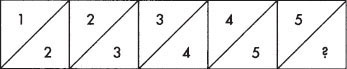a) 6

b) 7

c) 8

d) 9

2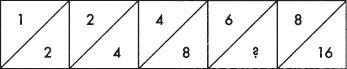a) 9

b) 10

c) 12

d) 14

3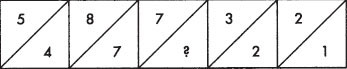a) 5

b) 6

c) 4

d) 3

4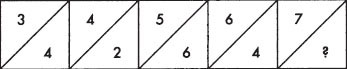a) 4

b) 5

c) 6

d) 8

5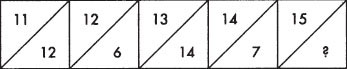b) 16

b) 18

c) 19

d) 20

6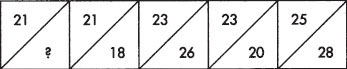a) 24

b) 19

c) 21

d) 25

7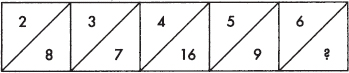a) 18

b) 20

c) 24

d) 26

8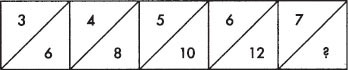a) 14

b) 16

c) 17

d) 18

9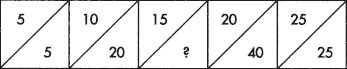a) 10

b) 15

c) 25

d) 50

10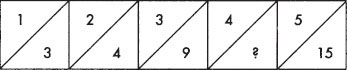a) 7

b) 8

c) 9

d) 12

11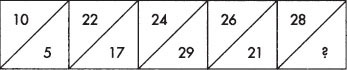a) 27

b) 29

c) 33

d) 35

12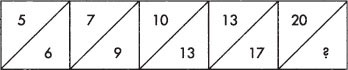a) 19

b) 21

c) 22

d) 25

13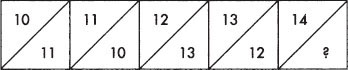a) 15

b) 11

c) 9

d) 7

14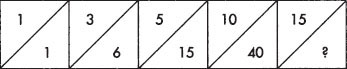a) 50

b) 55

c) 65

d) 75

15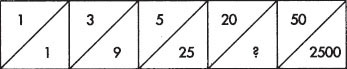a) 250

b) 400

c) 450

d) 1240

16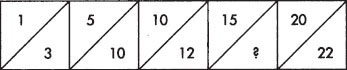a) 15

b) 18

c) 24

d) 30

17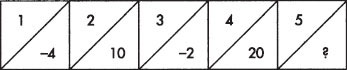a) −2

b) 0

c) 5

d) 40

18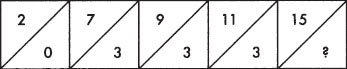a) 3

b) 5

c) 4

d) 6

19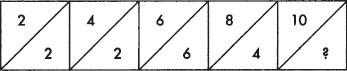a) 6

b) 8

c) 10

d) 12

20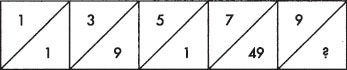a) 0

b) 1

c) 81

d) 90

## Numerical Tests 8 (Numerical Problem Solving)

These types of test are very commonly used in graduate and managerial selection, not least because they ask the test taker to apply their skills to deal with realistic problems in contexts that are expressly designed to approximate what actually happens in the workplace.

This test is almost a work sample. Pay particular attention to the fact that it requires no specialist financial or related knowledge. It samples the activities typically carried out by someone working with numerical information at a higher level, but does not need any more than a basic understanding of mathematical principles to complete. This is not at all like what one might expect from a marketing or accountancy qualifying exam – although it may look like one at first.

So please, if you see a test like this, do not be worried by it! At first glance the items look very complex and you will need to spend time understanding what is being asked of you. Do not worry if you don’t come up with the answer in a few seconds, that is not what the test is designed to allow you to do.

Конец ознакомительного фрагмента.